GFG App
Open AppBrowser
Continue

# Introduction to Data Structures

## WHAT IS DATA STRUCTURE?

In computer science, a data structure is a way of organizing and storing data in a computer program so that it can be accessed and used efficiently. Data structures provide a means of managing large amounts of data, enabling efficient searching, sorting, insertion, and deletion of data.

Data structures can be categorized into two types: primitive data structures and non-primitive data structures. Primitive data structures are the most basic data structures available in a programming language, such as integers, floating-point numbers, characters, and booleans. Non-primitive data structures are complex data structures that are built using primitive data types, such as arrays, linked lists, stacks, queues, trees, graphs, and hash tables.

The choice of data structure for a particular task depends on the type and amount of data to be processed, the operations that need to be performed on the data, and the efficiency requirements of the program. Efficient use of data structures can greatly improve the performance of a program, making it faster and more memory-efficient. A data structure is a particular way of organizing data in a computer so that it can be used effectively. The idea is to reduce the space and time complexities of different tasks.

The choice of a good data structure makes it possible to perform a variety of critical operations effectively. An efficient data structure also uses minimum memory space and execution time to process the structure.

### Data structures are an essential concept in computer science and programming. Here are some reasons why they are important:

1. Efficient data processing: Data structures provide a way to organize and store data in a way that allows for efficient retrieval, manipulation, and storage of data. For example, using a hash table to store data can provide constant-time access to data.
2. Memory management: Proper use of data structures can help to reduce memory usage and optimize the use of resources. For example, using dynamic arrays can allow for more efficient use of memory than using static arrays.
3. Code reusability: Data structures can be used as building blocks in various algorithms and programs, making it easier to reuse code.
4. Abstraction: Data structures provide a level of abstraction that allows programmers to focus on the logical structure of the data and the operations that can be performed on it, rather than on the details of how the data is stored and manipulated.
5. Algorithm design: Many algorithms rely on specific data structures to operate efficiently. Understanding data structures is crucial for designing and implementing efficient algorithms.

Overall, data structures are essential for managing and manipulating data in an efficient and effective way. They are a fundamental concept in computer science and are used extensively in programming and software development.

The structure of the data and the synthesis of the algorithm are relative to each other. Data presentation must be easy to understand so the developer, as well as the user, can make an efficient implementation of the operation.
Data structures provide an easy way of organizing, retrieving, managing, and storing data. Here is a list of the needs for data.

• Efficient data access and manipulation: Data structures enable quick access and manipulation of data. For example, an array allows constant-time access to elements using their index, while a hash table allows fast access to elements based on their key. Without data structures, programs would have to search through data sequentially, leading to slow performance.
• Memory management: Data structures allow efficient use of memory by allocating and deallocating memory dynamically. For example, a linked list can dynamically allocate memory for each element as needed, rather than allocating a fixed amount of memory upfront. This helps avoid memory wastage and enables efficient memory management.
• Code reusability: Data structures can be reused across different programs and projects. For example, a generic stack data structure can be used in multiple programs that require LIFO (Last-In-First-Out) functionality, without having to rewrite the same code each time.
• Optimization of algorithms: Data structures help optimize algorithms by enabling efficient data access and manipulation. For example, a binary search tree allows fast searching and insertion of elements, making it ideal for implementing searching and sorting algorithms.
• Scalability: Data structures enable programs to handle large amounts of data effectively. For example, a hash table can store large amounts of data while providing fast access to elements based on their key.

## Classification/Types of Data Structures:

Data structures can be classified into two main types: primitive data structures and non-primitive data structures.

Primitive data structures: These are the most basic data structures and are usually built into programming languages. Examples include:
Integer
Float
Character
Boolean
Double
Void
Non-primitive data structures: These are complex data structures that are built using primitive data types. Non-primitive data structures can be further categorized into the following types:
Arrays: A collection of elements of the same data type, stored in contiguous memory locations.

Linked lists: A collection of elements that are connected by links or pointers.

Stacks: A collection of elements that follow the Last-In-First-Out (LIFO) principle.

Queues: A collection of elements that follow the First-In-First-Out (FIFO) principle.

Trees: A hierarchical data structure consisting of nodes connected by edges.

Graphs: A non-linear data structure consisting of nodes and edges.

Hash tables: A data structure that stores data in an associative manner using a hash function.

Heaps: A specialized tree-based data structure that satisfies the heap property.

Tries: A tree-like data structure used to store associative arrays where the keys are strings.

Sets: A collection of unique elements.

Maps: An abstract data type that stores key-value pairs.

The choice of data structure depends on the problem to be solved and the operations to be performed on the data. Different data structures have different strengths and weaknesses and are suitable for different scenarios. Understanding the different types of data structures and their characteristics is important for efficient algorithm design and implementation.

1.     Linear Data Structure
2.     Non-Linear Data Structure.

### Linear Data Structure:

• A linear data structure is a type of data structure in which data elements are arranged in a sequential order, and each element has a unique predecessor and successor, except for the first and last elements. Linear data structures are one-dimensional and can be traversed sequentially from the first to the last element.
• Elements are arranged in one dimension ,also known as linear dimension.
• Example: lists, stack, queue, etc.

### Non-Linear Data Structure

• A Non-linear data structure is a type of data structure in which data elements are not arranged in a sequential order, and each element may have one or more predecessors and successors. Non-linear data structures can represent complex relationships between data elements, such as hierarchies, networks, and graphs.
• Elements are arranged in one-many, many-one and many-many dimensions.
• Example: tree, graph, table, etc.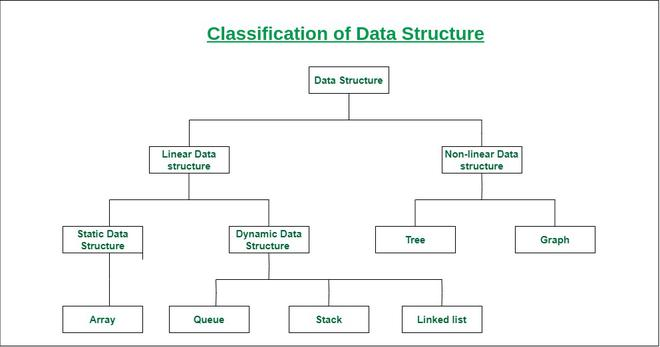## Most Popular Data Structures:

1. Array: An array is a collection of data items stored at contiguous memory locations. The idea is to store multiple items of the same type together. This makes it easier to calculate the position of each element by simply adding an offset to a base value, i.e., the memory location of the first element of the array (generally denoted by the name of the array).2. Linked Lists: Like arrays, Linked List is a linear data structure. Unlike arrays, linked list elements are not stored at a contiguous location; the elements are linked using pointers.3. Stack: Stack is a linear data structure which follows a particular order in which the operations are performed. The order may be LIFO(Last In First Out) or FILO(First In Last Out). In stack, all insertion and deletion are permitted at only one end of the list.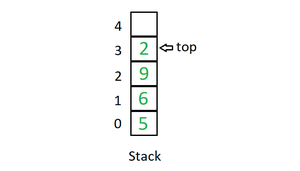Mainly the following three basic operations are performed in the stack:

• Initialize: Make a stack empty.
• Push: Adds an item in the stack. If the stack is full, then it is said to be an Overflow condition.
• Pop: Removes an item from the stack. The items are popped in the reversed order in which they are pushed. If the stack is empty, then it is said to be an Underflow condition.
• Peek or Top: Returns top element of the stack.
• isEmpty: Returns true if the stack is empty, else false.

4. Queue: Like Stack, Queue is a linear structure which follows a particular order in which the operations are performed. The order is First In First Out (FIFO). In the queue, items are inserted at one end and deleted from the other end. A good example of the queue is any queue of consumers for a resource where the consumer that came first is served first. The difference between stacks and queues is in removing. In a stack we remove the item the most recently added; in a queue, we remove the item the least recently added.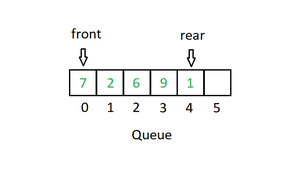Mainly the following four basic operations are performed on queue:

• Enqueue: Adds an item to the queue. If the queue is full, then it is said to be an Overflow condition.
• Dequeue: Removes an item from the queue. The items are popped in the same order in which they are pushed. If the queue is empty, then it is said to be an Underflow condition.
• Front: Get the front item from the queue.
• Rear: Get the last item from the queue.

5. Binary Tree: Unlike Arrays, Linked Lists, Stack and queues, which are linear data structures, trees are hierarchical data structures. A binary tree is a tree data structure in which each node has at most two children, which are referred to as the left child and the right child. It is implemented mainly using Links.

A Binary Tree is represented by a pointer to the topmost node in the tree. If the tree is empty, then the value of root is NULL. A Binary Tree node contains the following parts.

```1. Data
2. Pointer to left child
3. Pointer to the right child```

6. Binary Search Tree: A Binary Search Tree is a Binary Tree following the additional properties:

• The left part of the root node contains keys less than the root node key.
• The right part of the root node contains keys greater than the root node key.
• There is no duplicate key present in the binary tree.

A Binary tree having the following properties is known as Binary search tree (BST).

7. Heap: A Heap is a special Tree-based data structure in which the tree is a complete binary tree. Generally, Heaps can be of two types:

• Max-Heap: In a Max-Heap the key present at the root node must be greatest among the keys present at all of its children. The same property must be recursively true for all sub-trees in that Binary Tree.
• Min-Heap: In a Min-Heap the key present at the root node must be minimum among the keys present at all of its children. The same property must be recursively true for all sub-trees in that Binary Tree.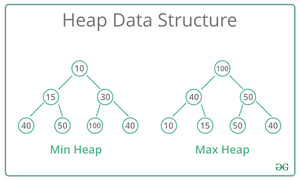8. Hashing Data Structure: Hashing is an important Data Structure which is designed to use a special function called the Hash function which is used to map a given value with a particular key for faster access of elements. The efficiency of mapping depends on the efficiency of the hash function used.

Let a hash function H(x) maps the value x at the index x%10 in an Array. For example, if the list of values is [11, 12, 13, 14, 15] it will be stored at positions {1, 2, 3, 4, 5} in the array or Hash table respectively.9. Matrix: A matrix represents a collection of numbers arranged in an order of rows and columns. It is necessary to enclose the elements of a matrix in parentheses or brackets.

A matrix with 9 elements is shown below.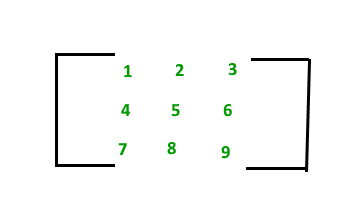10. Trie: Trie is an efficient information reTrieval data structure. Using Trie, search complexities can be brought to an optimal limit (key length). If we store keys in the binary search tree, a well-balanced BST will need time proportional to M * log N, where M is maximum string length and N is the number of keys in the tree. Using Trie, we can search the key in O(M) time. However, the penalty is on Trie storage requirements.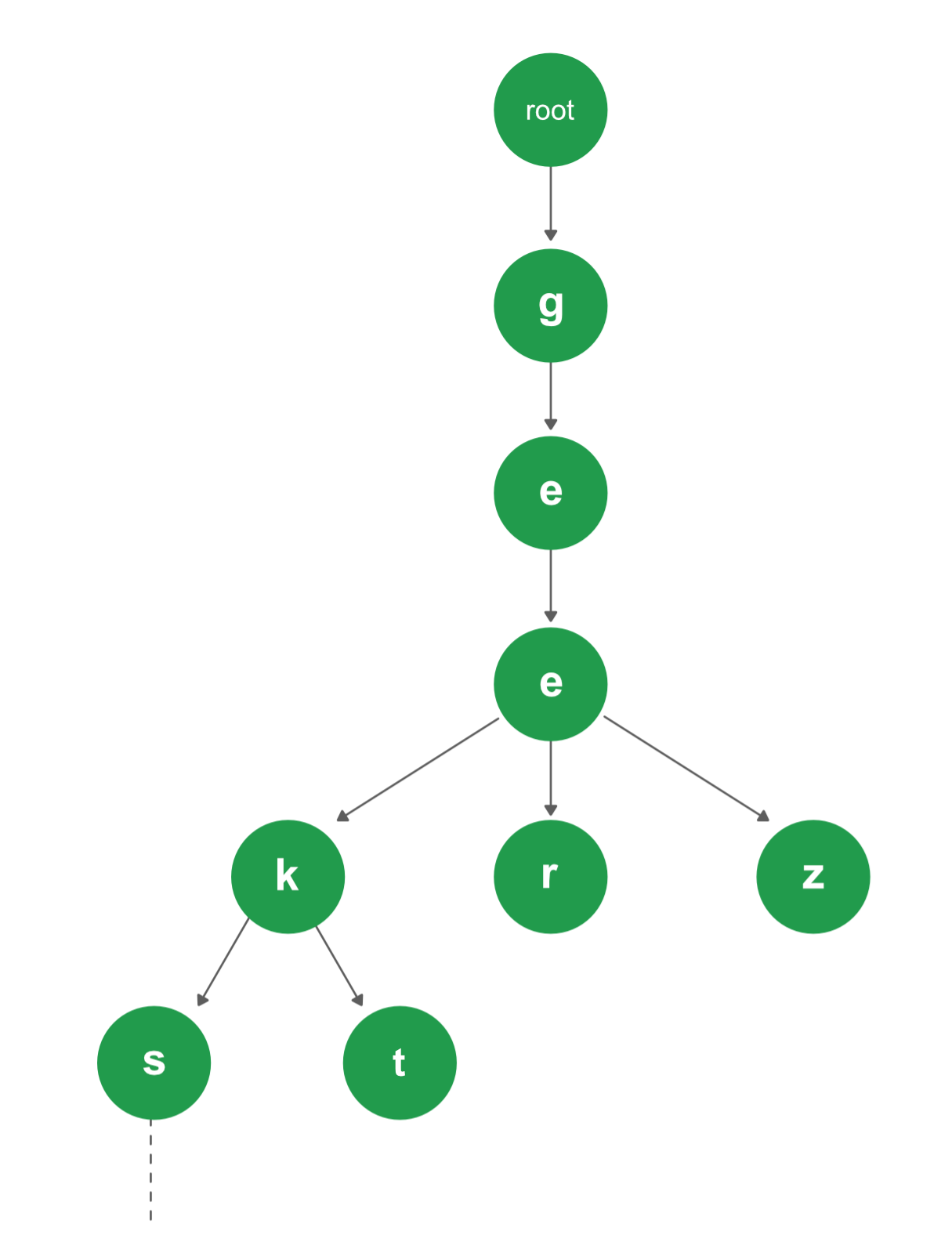## Applications of Data Structures:

• Arrays: Arrays are used to store a collection of homogeneous elements in contiguous memory locations. They are commonly used to implement other data structures, such as stacks and queues, and to represent matrices and tables.
• Linked lists: Linked lists are used to store a collection of heterogeneous elements with dynamic memory allocation. They are commonly used to implement stacks, queues, and hash tables.
• Trees: Trees are used to represent hierarchical data structures, such as file systems, organization charts, and network topologies. Binary search trees are commonly used to implement dictionaries and symbol tables.
• Graphs: Graphs are used to represent complex relationships between data elements, such as social networks, transportation networks, and computer networks. They are commonly used to implement shortest path algorithms and graph traversal algorithms.
• Hash tables: Hash tables are used to implement associative arrays, which store key-value pairs. They provide fast access to data elements based on their keys.
• Stacks: Stacks are used to store a collection of elements in a last-in-first-out (LIFO) order. They are commonly used to implement undo-redo functionality, recursive function calls, and expression evaluation.
• Queues: Queues are used to store a collection of elements in a first-in-first-out (FIFO) order. They are commonly used to implement waiting lines, message queues, and job scheduling.

My Personal Notes arrow_drop_up